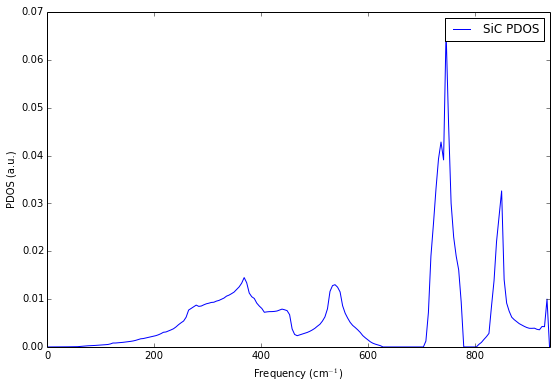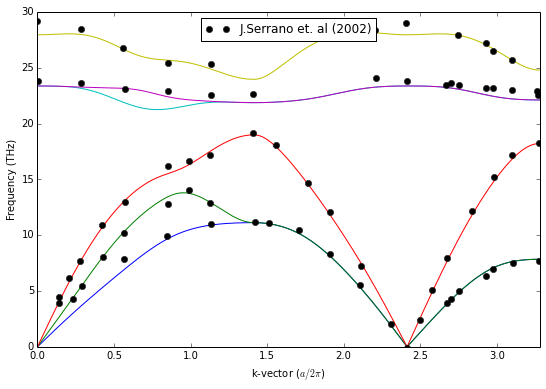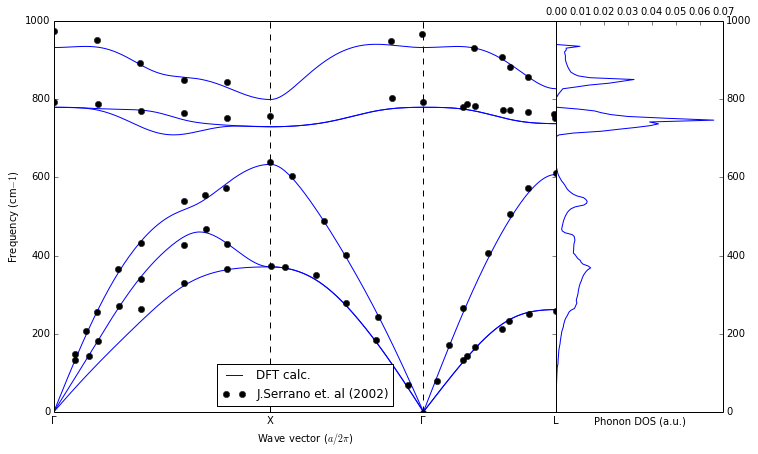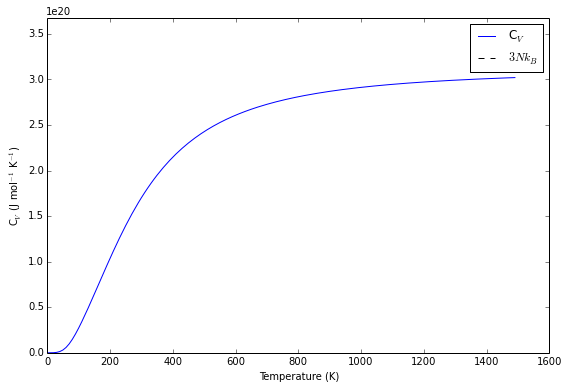Lattice dynamics calculations¶

The calculation of the lattice dynamics (i.e. the phonon density of states and phonon dispersion) requires fairly simple setup but is by itself time-consuming. It is best done using a multi-core machine (perhaps in some computing center). The intialization part is the same as in other tutorials (cell [1-3])

In :
# Import the basic libraries
from __future__ import division
# ASE system
import ase
from ase import Atom, Atoms
from ase import io
from ase.lattice.spacegroup import crystal

# Spacegroup/symmetry library
from pyspglib import spglib

# iPython utility function
from IPython.core.display import Image
In :
# Import the remote execution tools from the qe-util package
from qeutil import QuantumEspresso, RemoteQE

# Utility function for plotting standard phonon plots
from qeutil.analyzers import plot_phonons, get_thermodynamic_functions

Crystal setup¶

We use again a cubic SiC crystal. The setup of the crystal is the same as usual.

In :
# Stup the SiC crystal
# Create a cubic crystal with a spacegroup F-43m (216)
a=4.36
SiC = crystal(['Si', 'C'],
[(0, 0, 0), (0.25, 0.25, 0.25)],
spacegroup=216,
cellpar=[a, a, a, 90, 90, 90])

Calculator setup¶

For the lattice dynamics calculation the Quantum Espresso calculator needs more parameters then for the simple static calculation. Additional parameters required for the run are:

• qpts - grid [nqx,nqy,nqz] of the q-points defining sampling of the Brillouin zone
• qpath - a list of q-points forming a path in the reciprocal space along which the phonon dispersion will be calculated
• points - number of intervals between the points in the qpath (default=100)
• nkdos - a single number or a list of three numbers specifing a grid for integration of phonon density of states
• ndos - number of points along the energy axis for phonon DOS calculation

You do not need to specify all of this parameters right at the creation of the calculator but they must be provided by means of the set method or parameters to the get_ functions before the calculation.

In :
# Import actual access info for the remote execution from the external file.
import host
In :
# Create a Quantum Espresso calculator for our work.
# This object encapsulates all parameters of the calculation,
# not the system we are investigating.
qe=RemoteQE(label='SiC-LatDyn',
kpts=[4,4,4],    # Sampling grid in the reciprocal space for the electronic calculation
qpts=[4,4,4],    # Sampling grid in the reciprocal space for the phonon calculation
xc='pz',         # Exchange functional type in the name of the pseudopotentials
pp_type='vbc',   # Variant of the pseudopotential
pp_format='UPF', # Format of the pseudopotential files
ecutwfc=70,
pseudo_dir='../pspot',
use_symmetry=True,
procs=4)         # Use 4 cores for the calculation

# Check where the calculation files will reside on the local machine.
print qe.directory

# Assign the calculator to our system
SiC.set_calculator(qe)
calc/SiC-LatDyn._Vxxw4

Calculation procedure¶

The phonon dispersion is calculated in four stages:

1. Calculation of electronic structure of the crystal. This may be combined with the next step as it usually takes just a fraction of time of the next step.

2. Calculating second energy derivatives with respect to on the displacement of atoms and derivation of real-space force constants for the crystal. This is the most time consuming part of the calculation. It is split-off into a separate task and may take substantial amount of time. However this calculation needs to be performed only once for any given grid in the reciprocal space (qpts).

3. Calculating fourier interpolation of the dynamical matrix of the crystal for a set of points in the reciprocal space. Usually this is some path connecting high symmetry points in the Brillouin zone. This part of the calculation is fairly fast.

4. Integration of phonon modes over the whole Brillouin zone to obtain the phonon density of states (PDOS) function. If the specified integration grid is very dense (default is 15x15x15) this also may take few minutes.

All of these task are porformed in a different way than the calculations in other tutorials. Here we ask the calculator to compute one or more properties for a given structure. This mode allows for a more flexible control over the set of calculations performed, providing a way to avoid unecesary re-calculations. The drawback of this mode is an absence of automatic logic directing the ordering and requirement of the calculation, thus the responsibility for the correct execution of the tasks falls onto the user. Below we combine steps 1 and 2, as well as 3 and 4.

In :
# Run the first two stages of the task
qe.calculate(SiC,['energy','d2'])

Frequencies/PDOS calculation.¶

In the next step we prepare and execute the calculation of normal modes (frequencies) over the Brillouin zone path and phonon density of states. The parameters for a given stage/calculation may be set directly before the run, and changed as many times as required. The points are specified in carthesian coordinates in units of $2\pi/a$. You can find the coordinates of special points in the Brillouin zone using the Bilbao Crystallographic Server (the correct coordinates are in the "Conventional basis" column of the table.)

Note: At this moment the frequencies/PDOS are in cm$^{-1}$ - this may be changed in the future to better coordinate the calculator with the ASE system of units. Both are returned in the qe.results dictionary (see below).

In :
# Setup parameters for the second and third step of the procedure as well as for plotting.

# Several special points in the FCC lattice for conventional dispersion curves plot
# These particular points are selected to match the path used in experimental data.
G1=[0,0,0]
G2=[1,1,1]
X=[1,0,1]
L=[1/2,1/2,1/2]
W=[1/2,1/4,3/4]

# Set the path along which we would like to plot the phonon dispersion curves
qpath=array([G1,X,G2,L])
# Name the special points for plotting
qpname=[u'Γ','X',u'Γ','L']

# Put the parameters into the calculator
qe.set(qpath=qpath)       #   The path in the brillouin zone
qe.set(points=300);       #   Number of plot points between the special points along the dispersion curves
qe.set(nkdos=[15,15,15])      # Sampling grid in the reciprocal space for the PDOS integration
qe.set(ndos=200);              # Number of data points alog dos curve
Out:
{}
In :
# Step 3 and 4
qe.calculate(SiC,['phdos','frequencies'])

Plotting¶

One can plot directly the results of the calculations from the results['phdos'] and results['frequencies'] arrays. They are organized in the standard way. However producing a nice, standard plot of dispersion+PDOS together along the path may be a tedious tast. The qe-util library provides a utility function: plot_phonons which greatly simplifies making such plots. Here we will plot the results first the "pedestrian" way and then use the utility function.

The experimental data after J. Serrano, et al.; Appl. Phys. Lett. 80 (2002) 4360–4362.

In :
# PDOS
figsize(9,6)
plot(qe.results['phdos'],qe.results['phdos'],label='SiC PDOS');
xlim(0,max(qe.results['phdos']))                    # Limit the x-axis
xlabel('Frequency (cm$^{-1}$)');                     # Put proper description on axis
ylabel('PDOS (a.u.)')
legend();In :
# Phonon dispersion. Units transformed to THz.
# Conversion factor from inverse cm to THz
cminv2Thz=1/33.3564

# Plot the frequencies
for b in qe.results['frequencies'][1:]:
plot(qe.results['frequencies'], b*cminv2Thz, '-')

xlim(0,max(qe.results['frequencies']))
ylim(0,ylim())
xlabel('k-vector ($a/2\\pi$)')
ylabel('Frequency (THz)');

# Read in and plot the experimental data
# Due to the x-axis scalling in the data we need to scale and shift the x-axis for each segment.

# Plot the segments
plot(ex1*sqrt(2),ex1*cminv2Thz,'ok',label='J.Serrano et. al (2002)')
plot(ex2-1+sqrt(2),ex2*cminv2Thz,'ok')
plot(1+sqrt(2)+(ex3-2)*sqrt(3),ex3*cminv2Thz,'ok');

# Put the legend
legend(loc='upper center');The utility function provides many hooks for customisation of the plot and returns two handles to the two parts of the plot, so the user is free to furher manipulate the plot. Below is just an example of a typical presentation. There are many other variants possible. Furthermore if the flexibility of the function is not enough you can always copy its definition (the source is in the qeutil/analyzers.py file) and prepare your own variant.

In :
# Now use the utility function. This time we leave the frequencies in cm^-1 as is customary in experiment.
figsize(12,7)
ax1, ax2=plot_phonons(qe.results['frequencies'],qe.results['phdos'],qpath=qpath,qpname=qpname, label='DFT calc.')
ax2.set_xlabel('Wave vector ($a/2\\pi$)')
ax2.set_ylabel('Frequency (cm$-1$)')

ax1.set_xlabel('Phonon DOS (a.u.)')

# Plot the segments
plot(ex1*sqrt(2),ex1,'ok',label='J.Serrano et. al (2002)')
plot(ex2-1+sqrt(2),ex2,'ok')
plot(1+sqrt(2)+(ex3-2)*sqrt(3),ex3,'ok');
legend(loc='lower center');Thermodynamic functions¶

The lattice vibrations and their dependence on external conditions (temperature, pressure, volume) determine the thermodynamic properties of the crystal. In particular, having the PDOS function for the crystal one can determine the heat capacity by simple integration over the whole range of energies using PDOS as a weighting factor. The analyzers module provides the function get_thermodynamic_functions which calculates the appropriate integrals for a given range of temperatures. The function returns the phonon contribution to the free energy and heat capacity as a function of temperature. The returned $C_V$ is in J mol$^{-1}$ K$^{-1}$

In :
# Calculate free energy (phonon contribution) and heat capacity as a function of temperature
tfun=get_thermodynamic_functions(qe.results['phdos'],Tmax=1500,Tstep=10)
In :
# Get the physical constants

# Plot the Cv
figsize(9,6)
plot(tfun,tfun,label='C$_V$')
xlabel('Temperature (K)')
ylabel('C$_V$ (J mol$^{-1}$ K$^{-1}$)')
xmin,xmax=xlim()

# 3Nk_B - High temperature thermodynamic limit for C_v
hlines(3*2*Boltzmann*Avogadro,xmin,xmax,linestyles='dashed',label='$3Nk_B$')
xlim(xmin,xmax)
ylim(0,1.05*ylim())
legend();In :